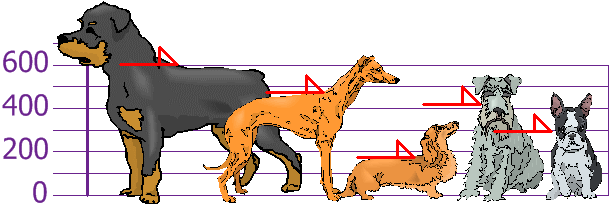# Variance

## What is Variance in Statistics?

Variance meaning – It is a measure of how data points differ from the mean. According to layman’s terms, it is a measure of how far a set of data( numbers) are spread out from their mean (average) value.

For the purpose of solving questions, it is,

Var (X) = E[ ( X – $\mu$))2]

Put into words; this means that variance is the expectation of the deviation of a random set of data from its mean value, squared. Here,

“µ” is equal to E(X) so the above equation may also be expressed as,

Var(X) = E[(X – E(X)2)]

Var(X) = E[ X2 -2X E(X) +(E(X))2]

Var(X) = E(X2) -2 E(X) E(X) + (E(X))2

Var(X) = E(X2) – (E(X))2

Now let’s have a look at the relationship between Variance and Standard Deviation.

## Variance Standard Deviation

Standard deviation is the positive square root of the variance. The symbols σ and S are used correspondingly to represent population and sample standard deviations.

Standard Deviation is a measure of how spread out the data is. Its formula is simple; it is the square root of the variance for that data set. It’s represented by the Greek symbol sigma (σ ).

### Formulas

The corresponding formulas are hence,

Population standard deviation σ = $\sqrt{\frac{\sum (X-\mu )^{2}}{N}}$ and

Sample standard deviation S = $\sqrt{\frac{\sum (X-\overline{X})^{2}}{n-1}}$

### Variance Properties

The variance, var(X) of a random variable X has the following properties.

1. Var(X + C) = Var(X), where C is a constant.
1. Var(CX) = C2.Var(X), where C is a constant.
2. Var(aX + b) = a2.Var(X), where a and b are constants.
3. If X1, X2,……., Xn are n independent random variables, then

Var(X1 + X2 +……+ Xn) = Var(X1) + Var(X2) +……..+Var(Xn).

### Learning Variance Concept with Example

The concepts mentioned above sound a little boring don’t they? The concept of statistics often appears this way since it means dealing with large volumes of data; It is essential that you, as a student, understand that these are not just numbers; If read properly, it tells you a story.  So let’s take a fun example of how to calculate variance in everyday life situation:Let’s say the heights at their shoulders (in mm) are 610, 450, 160, 420, 310.

Mean and Variance are interrelated. The first step is finding the mean which is done as follows,

Mean = ( 610+450+160+420+310)/ 5 = 390

So the mean average is 390 mm. Let’s plot this on the chart.

To calculate the Variance, compute the difference of each from the mean, square it and find then find the average once again.

So for this particular case the variance is :

=  (2202 + 602 + (-230)2 +302 + (-80)2)/5

= (48,400 + 3,600 + 52,900 + 900 + 6,400)/5

Final answer : Variance = 23,700

## Variance Example

Example: Find the variance of the numbers 3, 8, 6, 10, 12, 9, 11, 10, 12, 7.

Solution:

Step 1: Compute the mean of the 10 values given.

x = (3+8+6+10+12+9+11+10+12+7) / 10 = 88 / 10 = 8.8

Step 2: Make a table with three columns, one for the X values, the second for the deviations and the third for squared deviations.

 Value X X – $\bar{X}$ $(X-\bar{X})^2$ 3 -5.8 33.64 8 -0.8 0.64 6 -2.8 7.84 10 1.2 1.44 12 3.2 10.24 9 0.2 0.04 11 2.2 4.84 10 1.2 1.44 12 3.2 10.24 7 -1.8 3.24 Total 0 73.6

Step 3:

As the data is not given as sample data we use the formula for population variance.

σ2 = $\frac{\sum (X-\bar X )^{2}}{N}$

(Here $\mu = \bar X$)

= 73.6 / 10

= 7.36

Stay tuned with Byju’s to learn more about Covariance Formula and other maths concepts with the help of interactive videos.